## Parallelogram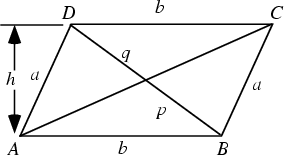A Quadrilateral with opposite sides parallel (and therefore opposite angles equal). A quadrilateral with equal sides is called a Rhombus, and a parallelogram whose Angles are all Right Angles is called a Rectangle.

A parallelogram of base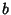and height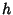has Area(1)

The height of a parallelogram is(2)

and the Diagonals are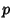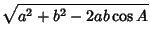(3)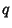(4)(5)

(Beyer 1987).

The Area of the parallelogram with sides formed by the Vectors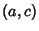and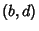is(6)

Given a parallelogram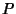with areaand linear transformation, the Area of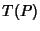is(7)As shown by Euclid,if lines parallel to the sides are drawn through any point on a diagonal of a parallelogram, then the parallelograms not containing segments of that diagonal are equal in Area (and conversely), so in the above figure,(Johnson 1929).

See also Diamond, Lozenge, Parallelogram Illusion, Rectangle, Rhombus, Varignon Parallelogram, Wittenbauer's Parallelogram

References

Beyer, W. H. (Ed.) CRC Standard Mathematical Tables, 28th ed. Boca Raton, FL: CRC Press, p. 123, 1987.

Johnson, R. A. Modern Geometry: An Elementary Treatise on the Geometry of the Triangle and the Circle. Boston, MA: Houghton Mifflin, p. 61, 1929.